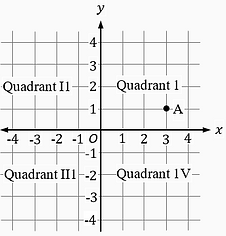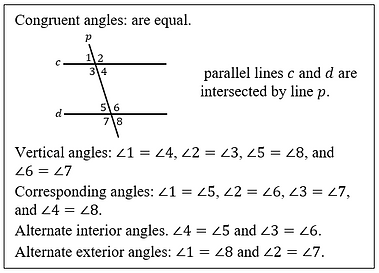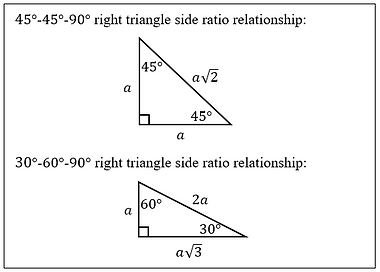Things to remember for the SAT math sections

Some general terms and what they refer to

xy-plane and ordered pair

xy-plane refers to a coordinate system that has a horizontal x-axis and a vertical y-axis. See figure below. The y-axis is perpendicular to the x-axis. The point where the two axes meet is known as the origin.

Each point in the coordinate system has a coordinate on the x-axis and a coordinate on the y-axis, collectively known as the ordered pair (x, y). For example, in the figure below, the x-coordinate of point A is 3 and the y-coordinate is 1. The ordered pair is written as (3, 1). The coordinates of origin are (0, 0).

Since every point of a line or a curve graph is plotted on the x- and y-axes, the term is used in questions to logically represent a line or a curve on a graph.The four sections formed by the intersecting x- and the y-axes in the xy-plane are known as quadrants. See figure above. They are numbered 1 to 4 in the counter-clockwise direction starting from the upper right quadrant. The quadrant numbers are denoted by Roman numerals.

Line segment

A line segment is a part of a line that has two distinct and finite end points. For example, sides of a triangle or a square or any other geometric figure that have distinct end points.

Variable

A variable is a placeholder for a number whose value is not known. It is denoted by an alphabet. For example, in 5x - 7, x is a variable. The value given to x will determine the value of 5x - 7.

Constant

A constant is a static number in an equation. For example, in the equation 2y = 5x - 7, the numbers 2, 5, and 7 are constants and x and y are variables. Their values will not change in the equation.

Sometimes a constant may be denoted by an alphabet in an equation. A question that has an alphabet as a constant will specifically call out the constant. For example, in the equation 2y = 5x - a, a is a constant.

Coefficient

A coefficient is a constant number multiplied by a variable. For example, in the equation 2y = 5x - 7, the constant 2 is the coefficient of the variable y and the constant 5 is the coefficient of the variable x.

Every variable has a coefficient. If a number is not given in front of the variable, then the value of the variable is 1 since 1 multiplied by x is x. For example, in the equation 2y = x - 7, the coefficient of the variable x is 1.

Term

A term is referred to a number, a variable, several variables multiplied together or a number and several variables multiplied together. For example, in the equation 2xy + x + 7 =  y - 1, the terms on the left side of the equation are 2xy, x, and 7 and the terms on the right side of the equation are  y and 1.

Expression

The terms on either side of an equation are collectively known as an expression. For example, in the equation 2x + 7 = x - 1, the two expressions are 2x + 7 and x - 1. An expression can be comprised of multiple terms and can contain plus, minus, multiplication, or division operators.

The distinction between an expression and an equation is important to remember. An equation contains an equal sign that equates two equal expressions, one on the left side of the equal sign and one on the right side of the equal sign.

Tangent

When two geometric figure in the xy-plane touch at one point, they are tangent to each other. For example, two circles are tangent to each other when they touch at one point, a line is tangent to a circle when it touches the circle the one point, a line is tangent to the parabola when it touches the parabola at one point, and so on.

Algebra Flash Cards

Few words/phrases and what they elude to...more in the book

Linear relationship between two variables

This implies that the two variables are the coordinates of points on a line.

The above terms refer to the same thing in a question on polynomial/quadratic equation. They refer to the points on the x-axis where the graph of a function intersects or touches the x-axis.

x-coordinate of the x-intercept

y is 0 at the x-intercept. Hence, the coordinates of the x-intercept are (x, 0). is the x-coordinate of the x-intercept.

y-coordinate of the y-intercept

is 0 at the x-intercept. Hence, the coordinates of the y-intercept are (0, y). is the y-coordinate of the y-intercept.

True for all values of x

This implies that the equation or the system of equations in the question has infinitely many solutions.​

Minimum/Maximum appears as constants or coefficients

This phrase refers to the vertex of a parabola in a quadratic function. When the parabola opens downwards, it is referred to as the maximum. When the parabola opens upwards, it is referred to as the minimum.

Half/Double/increase or decrease by x% over a certain period

​These terms refer to exponential growth or decay. Increase is growth and decrease is decay.

Plausible average increase/decrease

This refers to the value of the constant associated with the unknown number in the given linear equation.

Lines and SlopeSolutions of linear system of equationsEquations of a parabolaFactor theorem and Remainder theoremPercentExponential growth and decayCompound interest rateAbsolute value linear inequalitiesGeometry, Trigonometry,  and Complex Numbers Flash CardsCongruent anglesSupplementary anglesArea of a circle and sector of a circleCircumference of a circle and length of an arcEquation of a circleArea of a triangleSpecial right trianglesRight triangle and Pythagorean theoremSquare and cubeRectangle and right rectangular prismArea of a trapezoidArea of a parallelogramVolume of a sphereVolume of a coneVolume of a right rectangular prismVolume of a right cylinderTrigonometry functionsTrigonometry functionsComplex numbers